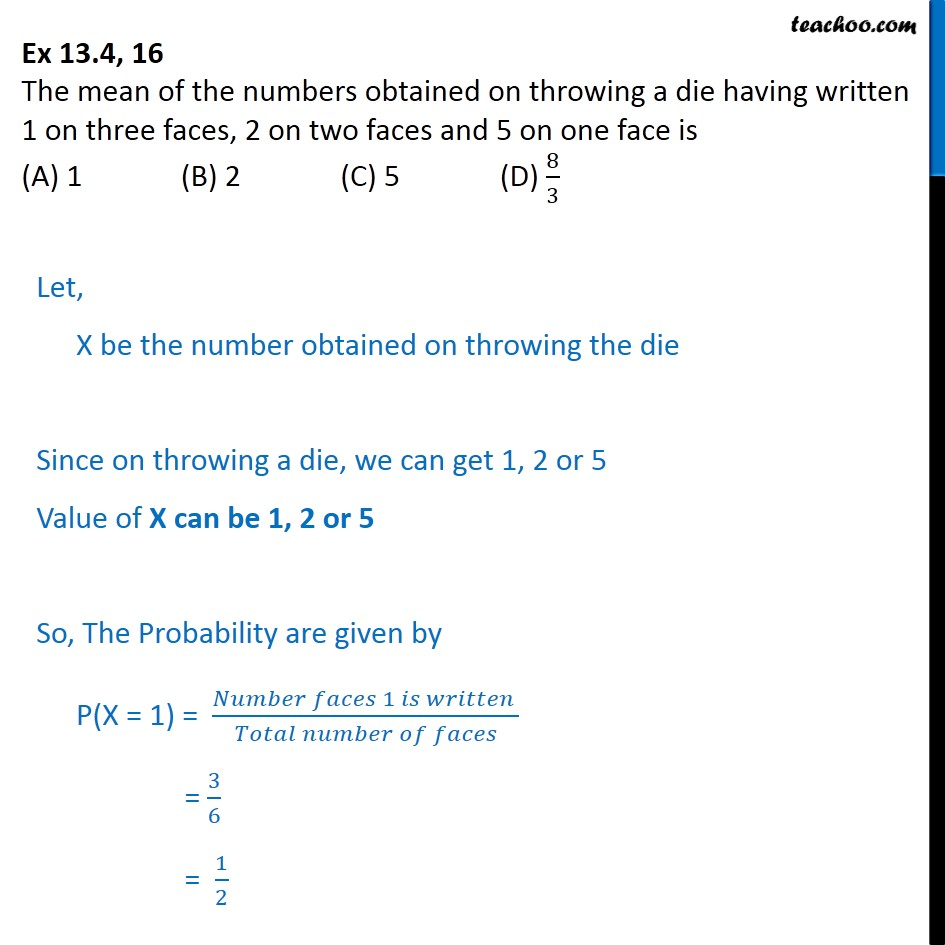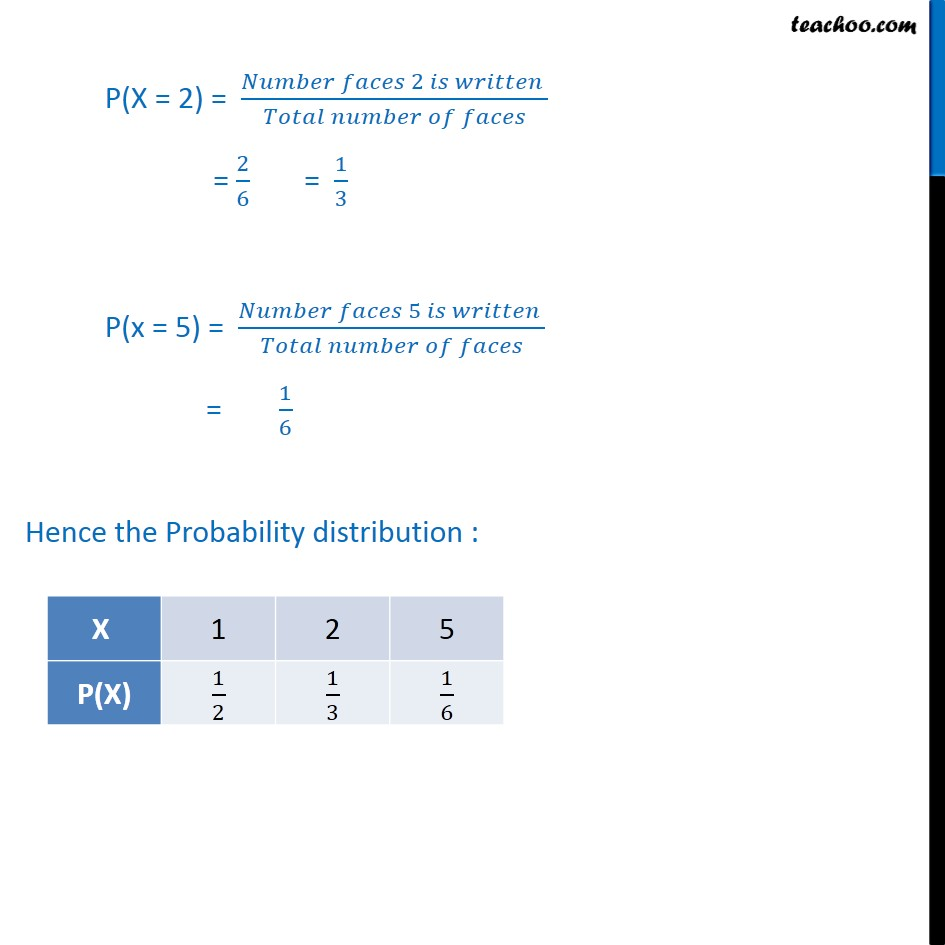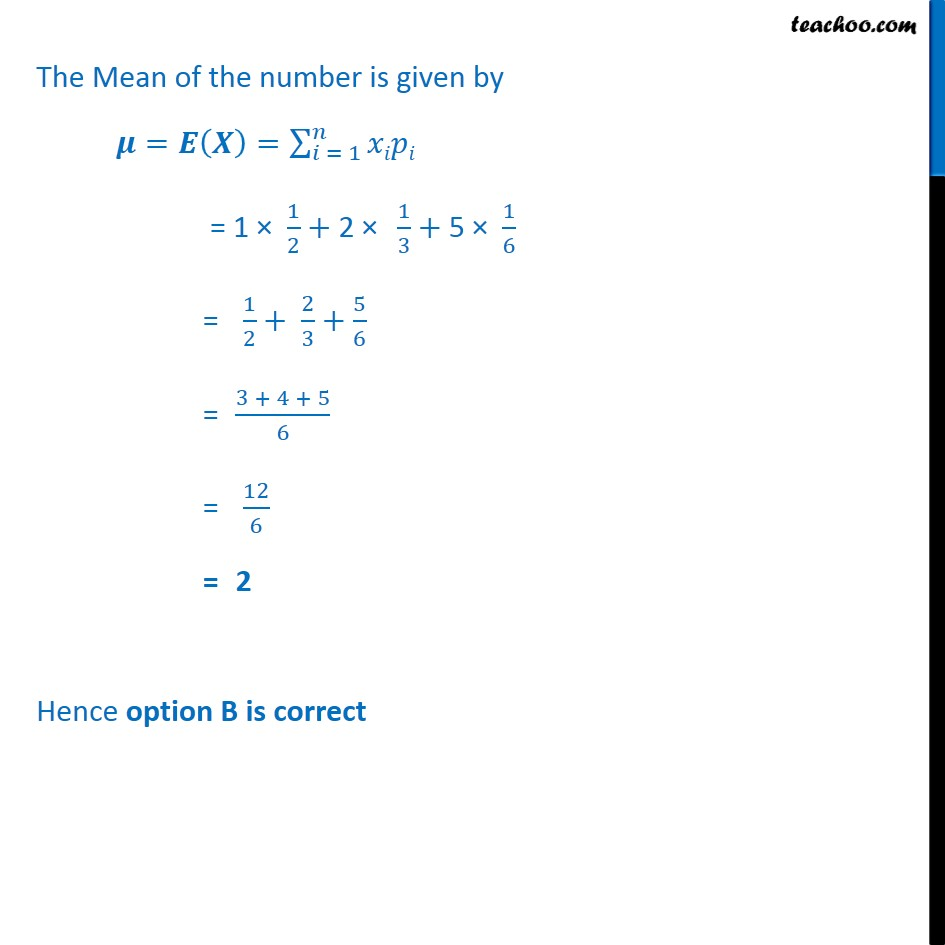Mean or Expectation of random variable

Chapter 13 Class 12 Probability (Term 2)
Concept wise### Transcript

Ex 13.4, 16 The mean of the numbers obtained on throwing a die having written 1 on three faces, 2 on two faces and 5 on one face is (A) 1 (B) 2 (C) 5 (D) 8﷮3﷯ Let, X be the number obtained on throwing the die Since on throwing a die, we can get 1, 2 or 5 Value of X can be 1, 2 or 5 So, The Probability are given by P(X = 1) = 𝑁𝑢𝑚𝑏𝑒𝑟 𝑓𝑎𝑐𝑒𝑠 1 𝑖𝑠 𝑤𝑟𝑖𝑡𝑡𝑒𝑛 ﷮𝑇𝑜𝑡𝑎𝑙 𝑛𝑢𝑚𝑏𝑒𝑟 𝑜𝑓 𝑓𝑎𝑐𝑒𝑠﷯ = 3﷮6﷯ = 1﷮2﷯ P(X = 2) = 𝑁𝑢𝑚𝑏𝑒𝑟 𝑓𝑎𝑐𝑒𝑠 2 𝑖𝑠 𝑤𝑟𝑖𝑡𝑡𝑒𝑛 ﷮𝑇𝑜𝑡𝑎𝑙 𝑛𝑢𝑚𝑏𝑒𝑟 𝑜𝑓 𝑓𝑎𝑐𝑒𝑠﷯ = 2﷮6﷯ = 1﷮3﷯ P(x = 5) = 𝑁𝑢𝑚𝑏𝑒𝑟 𝑓𝑎𝑐𝑒𝑠 5 𝑖𝑠 𝑤𝑟𝑖𝑡𝑡𝑒𝑛 ﷮𝑇𝑜𝑡𝑎𝑙 𝑛𝑢𝑚𝑏𝑒𝑟 𝑜𝑓 𝑓𝑎𝑐𝑒𝑠﷯ = 1﷮6﷯ Hence the Probability distribution : The Mean of the number is given by 𝝁=𝑬 𝑿﷯= 𝑖 = 1﷮𝑛﷮𝑥𝑖𝑝𝑖﷯ = 1 × 1﷮2﷯+2 × 1﷮3﷯+ 5 × 1﷮6﷯ = 1﷮2﷯+ 2﷮3﷯+ 5﷮6﷯ = 3 + 4 + 5﷮6﷯ = 12﷮6﷯ = 2 Hence option B is correct